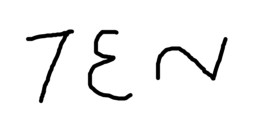# What is the largest number that you can write with just three digits?

1. What is the largest number that you can write with just three digits?

2.0
JThomp42posted 10 years ago

999 (9 to the 9th power of 9). Work out the second and third powers first (9*9*9*9*9*9*9*9*9 = 387420489.) We can therefore restate the sum as 9387420489

3. The biggest number that I can think of that has 3 digits is "999K". You didn't say that I couldn't add a letter.

4.0
paxwillposted 10 years ago

If we're allowed to use common symbols, my guess is (9!)^[(9!)^(9!)], which has around 10^2017526 digits according to Wolfram Alpha.

Without common symbols, my guess is 9 to the power of 9-to-the-9th (written as a tower of powers).  Since we can't format superscripts in the Q&A section, it's 9^(9^9) written in "linear" notation.  This works out to a number with 369,693,100 digits.

(Not sure if this is the same as JThomp's answer)

1. With symbols there's no limit: 9!!!^(9!!!^9!!!), 9!!!!!!^(9!!!!!!^9!!!!!!)....

2.0
paxwillposted 10 years agoin reply to this

Oh yeah, good point.

5. I've seen this puzzle before and once had an argument with a guy who insisted that the only correct answer was 999, as in nine hundred ninety-nine.  He said anything else was "an expression that evaluates to a number" but not actually a "number." Don't know if he really bought this or was just trying to be smug, lol.

6.0
calculus-geometryposted 10 years agoWith three digits the largest number I can write is "ten" using 7 = T, 3 = E, and 2 = N.

7. The largest number you can write doesn't depend on the actual digits, but the limitations of your available font size at hand; ha!  For example, if you use the Nazca Lines method, you could write some really large numbers, even if it was a mere 111...

8. All of these answers that use "9" assume that the number is written in Base 10. Another common counting system is hexadecimal, or base sixteen. Not using any other symbol, it's highest 3-digit number is FFF, which translates into decimal as 15^3+15^2+15, or 3375+225+15, or 3,615.

But, of course, theoretically, any base is possible, so that the number in three digits could be infinitely high.

I'm beginning to wonder what CWanamaker was thinking when he wrote the question that has so many answers?

1. Good thinking outside of the box.  I should have been more specific in my question...there is a wide range of interpretations and answers given.

2. Thanks, CWanamaker. I'd be really intrigued to see you post a new question, more precise, along the original lines of your inquiry.

9. It depends on what base you're talking about and whether or not you're talking about a positional numbering system. In hex, it would be FFF. In decimal, 999.

But let's say you have base 1,000,000,000,000,000, and the highest single digit is represented by the asterisk. Then the largest 3-digit number would be ***. Converting *** to decimal (scientific notation) would yield 1.0e45-1.

But then you could create a base 1.0e45. Say it's highest single digit is #. Then the largest 3-digit number would be ### = 1.0e135-1 (decimal).

So, as you can see, the largest 3-digit number in any base is the highest single digit in that base used 3 times (in a positional numbering system).

1. Thanks, Lonestar! You picked up on my idea of using different bases and explained it well.

working